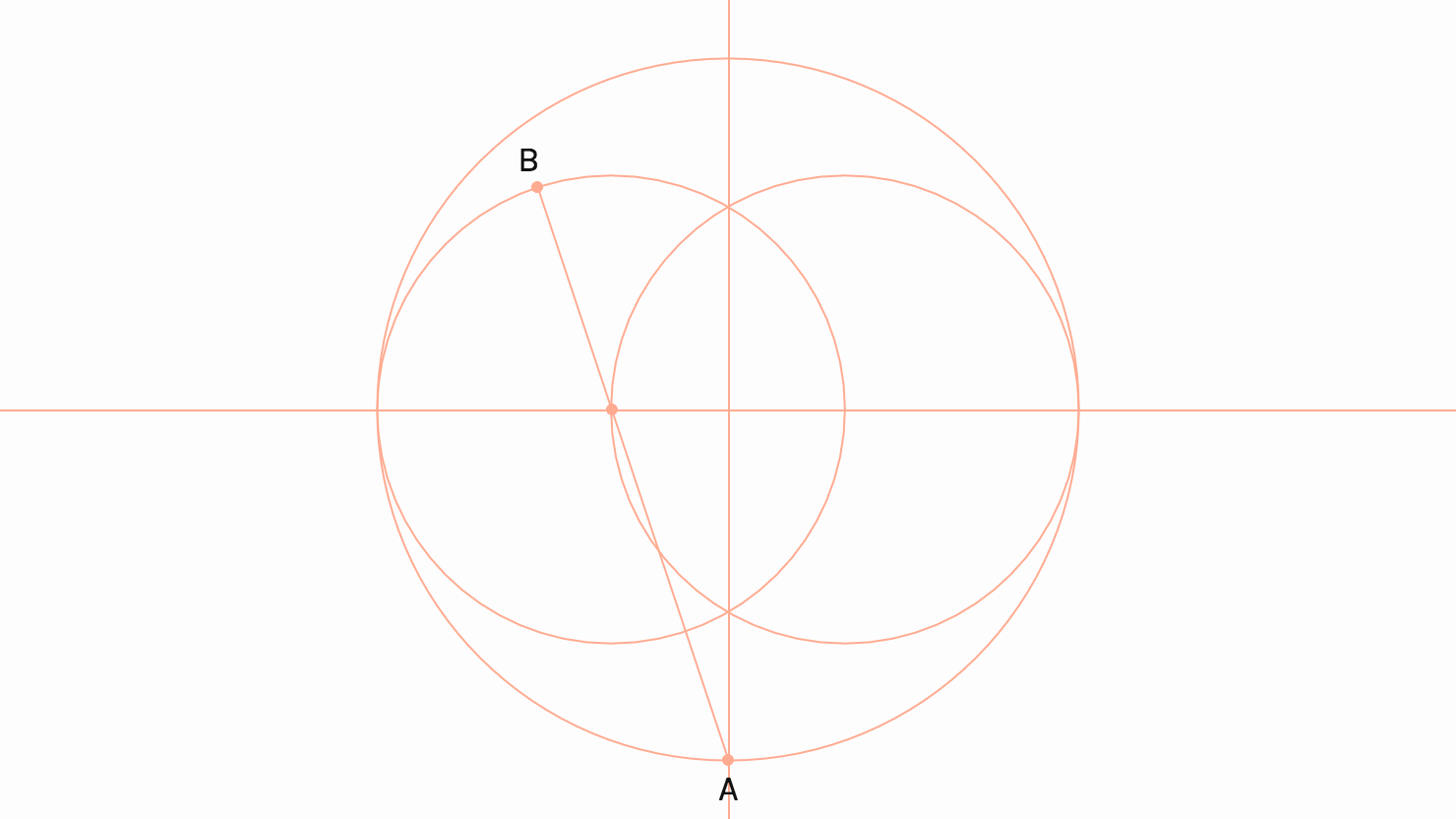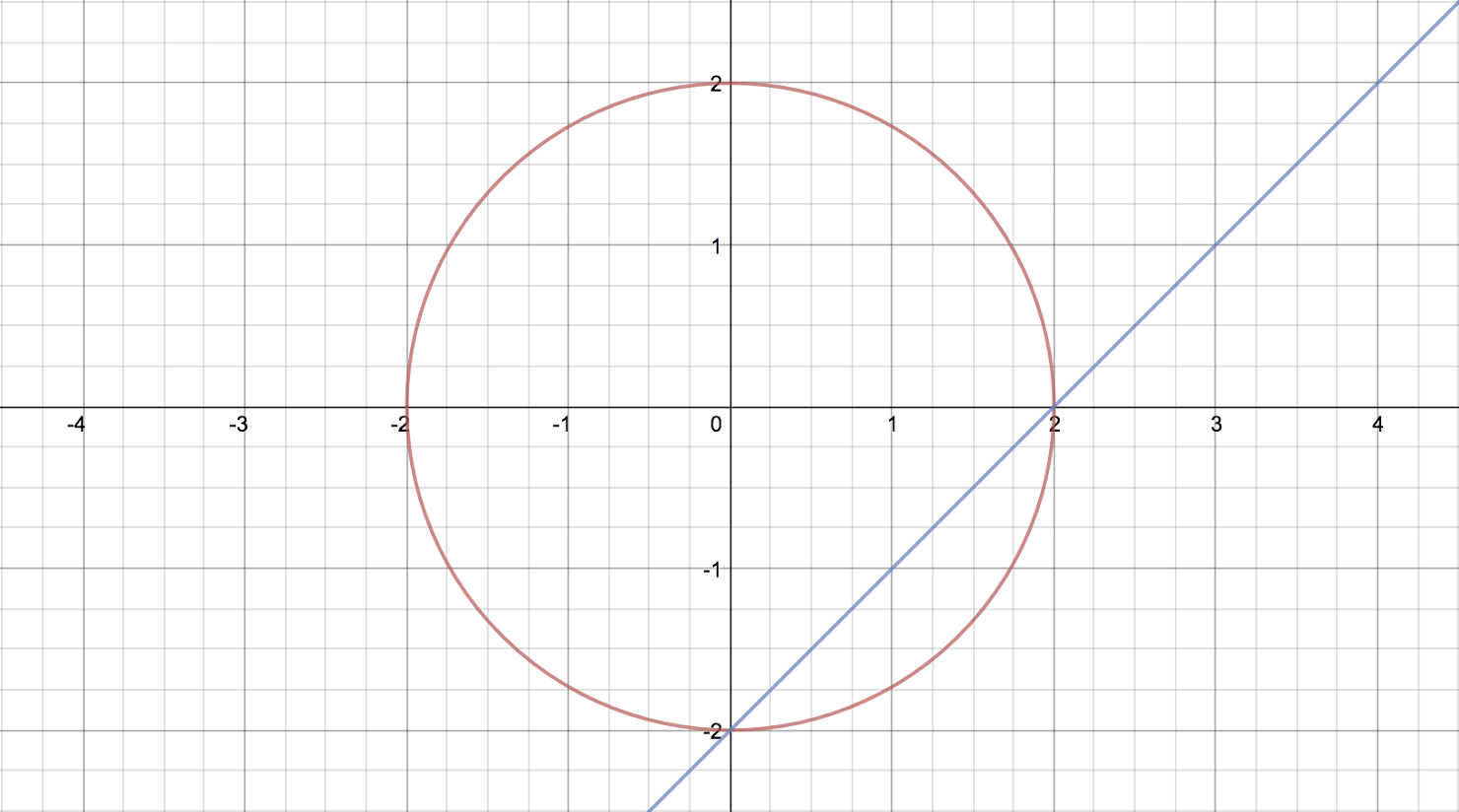For the previous post, I had to brush up on my math to construct Thom’s egg. The diagonal lines start from the point where the bottom arc intersects the y-axis (x = 0):If you were drawing this on paper, you could simply place your ruler along point A and the left circle centre and draw a line segment until you hit the circle. However, when drawing with p5.js you have to enter exact coordinates like this:

Point A is easily calculated. If the center of the sketch is (0, 0), the y-value of A is the (positive) radius of the bottom arc. Remember that the direction of the y-axis is reversed in computer graphics. To calculate point B, we have to algebraically figure out where the line segment starting from point A will intersect the left circle in the top left quadrant.

A circle can be defined as (x - h)^2 + (y - k)^2 = r^2, with radius r and (h, k) as its centre coordinate. A line can be defined as y = mx + n, where m is the slope or gradient and n is the y-intercept.

Before we go all out with the variables, let’s take a look at a basic example. Given r = 2 with centre (0, 0) and line y = x - 2, at which points will the line intersect with the circle?(x - 0)^2 + (y - 0)^2 = 2^2, simplify to:
x^2 + y^2 = 2^2

We can substitute y in the circle equation with x - 2, so we can solve for x:

x^2 + (x - 2)^2 = 2^2

Expand and combine like terms:

x^2 + (x - 2)(x - 2) = 2^2
x^2 + x^2 - 2x -2x + 4 = 2^2
2x^2 - 4x + 4 = 2^2
2x^2 - 4x + 4 - 2^2 = 0
2x^2 - 4x = 0

We now have a quadratic equation (ax^2 + bx - c = 0) that we can solve with the quadratic formula:

x = (-b +- \sqrt{b^2 - 4ac}) / (2a)

We first check if the equation has any solutions by calculating the discriminant:

D = b^2 - 4ac

There are 3 possible outcomes:

1. D > 0, there are two different solutions.
2. D = 0, there is one solution (or two solutions with the same value).
3. D < 0, there are no solutions.

In the case of a = 2, b = -4 and c = 0 there are 2 solutions. Let’s find out what they are:

x_1 = (4 + \sqrt{4^2 - 4 * 2 * 0}) / (2 * 2)
x_1 = 2
x_2 = (4 - \sqrt{4^2 - 4 * 2 * 0}) / (2 * 2)
x_2 = 0

This means that the line will intersect the circle at x_1 = 2 and x_2 = 0. Great! How can we put this to use in p5.js? Well, instead of solving this equation in multiple steps by hand, we can derive the a, b and c values directly from the circle (x - h)^2 + (y - k)^2 = r^2 and line y = mx + n equations and use these in the quadratic formula:

If you aren’t using p5.js, you can substitute the functions sq(x) and sqrt(x) with their native JavaScript counterparts: x * x and Math.sqrt(x).

In Thom’s egg, the slope and y-intercept values for the linear equation of the line segment can be inferred from two given points: the point where the bottom arc intersects the y-axis and the centre of the left circle.

There probably is another, more geometric way to calculate the length of the diagonal line segment in this construction, given the relative sizes of the constituent lengths, but the function that is described above will also be useful in other scenarios when the intersections cannot be inferred from other data.